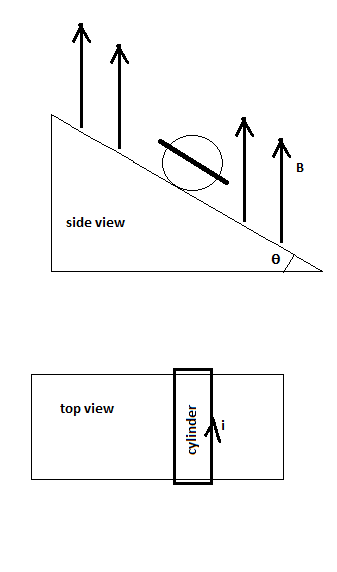# The figure shows a cylinder of mass 1.49 kg, radius 3.40 cm and length 9.03 cm with 130 turns of...

## Question:

The figure shows a cylinder of mass 1.49 kg, radius 3.40 cm and length 9.03 cm with 130 turns of wire wrapped around it lengthwise, so that the plane of the wire loop is parallel to the incline and contains the axis of the cylinder. What is the least current which while flowing through the loop will prevent the cylinder from rolling or sliding down the inclined plane in the presence of a vertical magnetic field of B = 0.28 T? The angle of inclination {eq}= 20.0^\circ {/eq}. The plane of the winding is parallel to the inclined plane. Assume that the wire loop has the same dimensions as the cylinder.## Electric current:

When the voltage difference is applied at two points in the circuit, the electron moves and carries the charges, these charges carried by the electrons in the circuit is termed as electric current.

Given data

• Mass of cylinder is, {eq}m = 1.49\;{\rm{kg}} {/eq}.
• Radius of cylinder is, {eq}R = 3.4\;{\rm{cm}} = 0.034\;{\rm{m}} {/eq} .
• Length of cylinder is, {eq}L = 9.03\;{\rm{cm}} = 0.0903\;{\rm{m}} {/eq} .
• Number of turns are, {eq}N = 130 {/eq} .
• Magnetic field intensity is, {eq}B = 0.28\;{\rm{T}} {/eq} .
• Inclination angle is, {eq}\theta = 20^\circ {/eq} .

The expression for the equilibrium of force in cylinder is,

{eq}F + ma = mg\sin \theta .............\left( 1 \right) {/eq}

Here, F is the static friction.

The expression the torque on cylinder due to friction force is,

{eq}T = F \times R..............\left( 2 \right) {/eq}

The expression for torque due to current is,

{eq}T = \mu \times B\sin \theta ...................\left( 3 \right) {/eq}

Here, {eq}\mu {/eq} is the magnetic moment.

The expression for magnetic moment is,

{eq}\mu = IAN................\left( 4 \right) {/eq}

Here, A is the horizontally projected area of cylinder.

The expression for horizontally projected area of cylinder is,

{eq}A = 2RL {/eq}

Substitute the values in equation (4).

{eq}\mu = 2IRLN {/eq}

Substitute the values in equation (3).

{eq}T = 2IRLN \times B\sin \theta ...............\left( 4 \right) {/eq}

From equation (2) and (4).

{eq}\begin{align*} 2IRLNB\sin \theta &= F \times R\\ 2ILNB\sin \theta &= F \end{align*} {/eq}

Substitute the value of F in equation (1).

{eq}2ILNB\sin \theta + ma = mg\sin \theta {/eq}

According to question the cylinder has to be at rest.

Then, {eq}a = 0 {/eq}

Substitute the values in above expression.

{eq}\begin{align*} 2ILNB\sin \theta + 0 &= mg\sin \theta \\ 2 \times I \times 0.0903 \times 130 \times 0.28 &= 1.49 \times 9.81\\ I &= 1.77\;{\rm{A}} \end{align*} {/eq}

Thus, the least current should be 1.77 A.